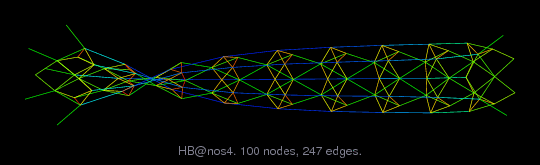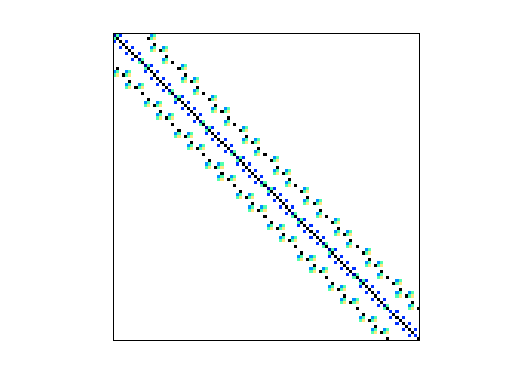Matrix: HB/nos4

Description: SYMMETRIC MATRIX OF BEAM STRUCTURE, NOVEMBER 1982.(undirected graph drawing)• Matrix group: HB
• download as a MATLAB mat-file, file size: 1 KB. Use UFget(220) or UFget('HB/nos4') in MATLAB.

 Matrix properties number of rows 100 number of columns 100 nonzeros 594 structural full rank? yes structural rank 100 # of blocks from dmperm 1 # strongly connected comp. 1 explicit zero entries 0 nonzero pattern symmetry symmetric numeric value symmetry symmetric type real structure symmetric Cholesky candidate? yes positive definite? yes

 author H. Simon editor I. Duff, R. Grimes, J. Lewis date 1982 kind structural problem 2D/3D problem? yes

 Ordering statistics: result nnz(chol(P*(A+A'+s*I)*P')) with AMD 632 Cholesky flop count 4.4e+03 nnz(L+U), no partial pivoting, with AMD 1,164 nnz(V) for QR, upper bound nnz(L) for LU, with COLAMD 767 nnz(R) for QR, upper bound nnz(U) for LU, with COLAMD 1,414

 SVD-based statistics: norm(A) 0.849138 min(svd(A)) 0.000537953 cond(A) 1578.46 rank(A) 100 sprank(A)-rank(A) 0 null space dimension 0 full numerical rank? yes

 singular values (MAT file): click here SVD method used: s = svd (full (A)) ; status: ok